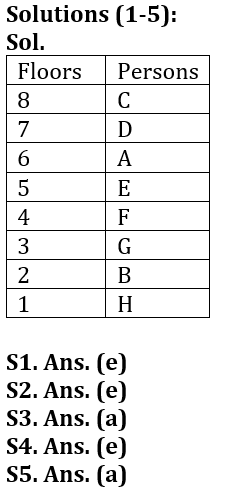Latest Banking jobs   »

# Reasoning Quiz For Bank of Baroda AO 2023-3rd March

Directions (1-5): Study the information carefully and answer the question given below.
There are eight persons A, B, C, D, E, F, G and H living on an eight-floor building (Ground floor is numbered 1 and topmost floor is number 8.) but not necessary in the same order. F lives on floor number 4. G lives on an odd number floor below 6th floor. D lives on one of the odd number floors but not below G’s floor. There is one person living between C and A who lives just above E. B lives on the floor immediate above H. C lives on a floor above A. Not more than two persons live between A and G.

Q1. On which of the following floor G lives?
(a) 4th
(b) 5th
(c) 7th
(d) 1st
(e) 3rd

Q2. How many persons live between D and C?
(a) One
(b) Two
(c) Three
(d) Four
(e) None

Q3. If all the persons are arranged alphabetically from top to bottom then how many persons remain (live) on same floor?
(a) One
(b) Two
(c) Three
(d) Four
(e) None

Q4. Four of the following five are alike in a certain way based on their arrangement find the one who does not belong to that group?
(a) B
(b) A
(c) F
(d) C
(e) G

Q5. If C is related to A, in the same way D is related to E, then following the same pattern, F is related to?
(a) B
(b) A
(c) E
(d) C
(e) None of these

Directions (6–10): Study the following arrangement carefully and answer the questions given below:

7 D 5 # A B 1 % K \$ 4 E J F € & 2 H I @ L 6 Q U © 9 M T 8 W

Q6. How many such symbols are there in the above arrangement each of which is immediately preceded by a vowel and also immediately followed by a number?
(a) None
(b) One
(c) Two
(d) Three
(e) Four

Q7. Four of the following five are alike in a certain way on the basis of their positions in the above arrangement and so form a group. Which is the one that does not belong to the group?
(a) K41
(b) &HF
(c) #B5
(e) LQI

Q8. Which of the following pairs of elements has the second element immediately followed by the first element?
(a) 5#
(b) MT
(c) \$4
(d) Q6
(e) @L

Q9. How many such numbers are there in the above arrangement, each of which is immediately followed by a symbol and also immediately preceded by a consonant?
(a) None
(b) One
(c) Two
(d) Three
(e) More than three

Q10. If all the symbols are removed, then which of the following element is 6th to the right of the element which is 10th from the left end?
(a) Q
(b) H
(c) 6
(d) L
(e) None of these

Directions (11-15): In these questions, relationship between different elements is shown in the statements. These statements are followed by two conclusions. Mark answer as
(a) If only conclusion I follows.
(b) If only conclusion II follows.
(c) If either conclusion I or II follows.
(d) If neither conclusion I nor II follows.
(e) If both conclusions I and II follow.

Q11. Statements: A≤M>G >K >D, M≤O< S, K<H
Conclusions: I. G < S II. A > D

Q12. Statements: B ≥ E >L<O>R=U
Conclusions: I. B<L II. U=L

Q13. Statements: X ≥ Q > F, A > F, R = M ≥ F
Conclusions: I. R > A II. X > F

Q14. Statements: D ≤ G > K ≥ R > T, G ≤ E > W, R < Y
Conclusions: I. W ≥ D II. Y > T

Q15. Statements: B ≥ K > G, M ≤ G, D = W ≥ G
Conclusions: I. D ≥ M II. K < W

SolutionsSolutions (6-10):
S6. Ans. (b)
S7. Ans. (c)
S8. Ans. (d)
S9. Ans. (c)
S10. Ans. (c)

Solutions (11-15):
S11. Ans.(a)
Sol. I. G < S (true) II. A > D (false)
S12. Ans.(d)
Sol. I. B<L (false) II. U=L (false)
S13. Ans.(b)
Sol. I. R > A (false) II. X > F (true)
S14. Ans.(b)
Sol. I. W ≥ D (false) II. Y > T (true)
S15. Ans.(a)
Sol. I. D ≥ M (true) II. K < W (false)## FAQs

### What is the selection process of Bank of Baroda AO 2023?

The selection process of Bank of Baroda AO consists of Online exam and Interview

#### Congratulations!Union Budget 2023-24: Free PDF